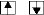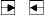Steps in the Numerical Solution

In either case, squaring the wave function would give a probability of infinity beyond the edges of the potential, a condition that we cannot accept. We need a wave function that goes to zero near the edges. Such a function must lie at an energy somewhere between the energies that gave these two unacceptable wave functions.

To get closer to the right wave function, you can use the arrow keys () to increase or decrease energy andto increase or decrease the increment in energy. Use the keys to obtain an acceptable wave function and its corresponding energy.